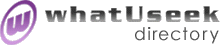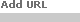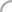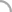SearchThe Directory The Webfor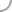SoftwareNew! Submit a site

Categories:
 MATLAB *(120)Mathcad *(11) Maple *(20)Mathematica *(38)

whatUseek Collection Sites (submit a site ):

MathSuite v.5.00 Official Website - MathSuite - This is the official website for MathSuite's Windows Software, that is a Powerful Calculus Environment and Matrixes Handling Engine.

whatUseek Directory Site Listings:

A Tutorial Introduction To AXIOM - By Martin N. Dunstan.

AXIOM - Symbolic solver complete with a high-level interactive language, user extensible library and visualisation tool for the manipulation of graphical output. For Unix and Windows.

Algebra Problem Solver - Personal Algebra Tutor - Enter your algebra problems and get instant step-by-step solutions with explanations.

Algebra Word Problem Tutor:Miss Lindquist - The world's first web-delivered self-improving intelligent tutoring system able to tutor students in writing expressions for algebra word problems.

CLICAL - A calculator type computer program for vectors, complex numbers, quaternions, bivectors, spinors, and multivectors in Clifford algebras.

CMAT - A matrix calculator program, written in C. Calculations can be performed on matrices with complex rational coefficients using exact arithmetic routines, as well as on matrices with elements mod p.

CoCoA - A special-purpose system for computations in Commutative Algebra.

Combinatorial Representation Theory (CREP) - CREP is designed to deal with categories whose morphism spaces are finite-dimensional over a field k. The main example of a category with this property is the category of finite-dimensional representations of an associative unital k-algebra.

Comparison of Polynomial-Oriented Computer Algebra Systems - By Robert H. Lewis and Michael Wester. Presented as a poster to the 1999 ISSAC Conference.

Computer Algebra Benchmarks - Collated by Computer Algebra Fachgruppe, Mannheim.

Computer Algebra Pages and Servers - A collection of links.

Computer Algebra and Problem Solving Environments - Abstract for Stanly Steinberg's article in "Comparative CAS Reviews and Philosophy", suggesting 6 ways in which computer algebra systems could be improved.

Derive for Windows - Compact, easy to use, reliable computer algebra software. It intelligently applies the rules of algebra, trigonometry, calculus and matrix algebra to solve a wide range of mathematical problems. This nonnumeric approach goes far beyond the capabilities of dedicated statistics packages and equation solvers that use only approximate numerical techniques.

Edu2000 Visual Algebra - Visual Algebra is designed to ease the difficulties many students experience during the transition from arithmetic into the world of algebra using visualization technology.

FRISCO - A Framework for Integrated Symbolic/Numeric Computation - The FRISCO project aims to develop highly efficient, versatile polynomial solvers for industrial users.

Felix - Computer algebra system for computation in commutative and non-commutative rings and modules.

Fermat - Computer algebra system that does arithmetic of arbitrarily long integers and fractions, symbolic calculations, graphics, and other numerical calculations. Free download. Documentation.

Free Complex Matrix and Vector classes - Two C++ classes: implementations of a matrix and a vector of complex numbers. Free to use for everyone.

GB and FGB - Software for solving algebraic equations: GB computes Groebner bases and FGB solves polynomial systems.

[ 1 2 3 ]Help build the largest human-edited directory on the web.About   Help   Content Filter   Terms   Privacy Policy© 2018 whatUseek